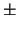Next: 6.1 Device Equation Damping Up: Dissertation Grasser Previous: 5.9 Thermal Simulation

# 6. Convergence and Damping

The system of equations which has to be solved for mixed-mode device simulation is non-linear and extremely sensitive to small changes in the solution variables. While the semiconductor equations are difficult to solve themselves the situation becomes even worse when using dynamic mixed-mode boundary conditions. To solve these equations the Newton method is used which is known to have quadratic convergence properties for an initial-guess sufficiently close to the final solution. However, such an initial-guess is hard to construct for both the distributed quantities inside the device and the circuit equations. Hence methods have to be found to enlarge the region of convergence to succeed even with a poor initial-guess. This is achieved by suitable damping schemes which will be discussed in the following sections. Damping schemes for the device equations will be discussed in Section 6.1 while Section 6.2 focuses on damping schemes for the circuit equations.

Especially important is a reliable method to obtain a DC operating point which is needed as a starting point for a transient analysis or a static transfer characteristic. Transient simulations are far better conditioned as the time derivatives provide main-diagonal entries and act as a natural damping. As the solution of the last timestep provides a good initial-guess it is normally possible to obtain convergence for a sufficiently small timestep. Although the conditioning of the equation system does not change for DC transfer analysis the last solution again provides a good initial-guess. In case the system fails to converge for a given step the step can normally be reduced in such a way to obtain convergence. Hence the following discussion will focus solely on DC operating point calculation.

To the best knowledge of the author no useful damping scheme for mixed-mode has been published so far. Only in  it was stated that the change of the node voltages was limited to a user-specified value which is in the range of 2 . VT. This is, as pointed out in the same paper, far from being optimal as it guarantees a large number of iterations for larger supply voltages as is the case for some of the circuits simulated in this thesis. E.g., for the output stage of an OpAmp as shown in Fig. 6.8 the supply voltages are15 V, hence it takes at least 15/0.05 = 300 iterations to build up the supply voltages without even considering the effect of non-linearities. Furthermore it is stated in  that a solution can only be obtained for an initial-guess as close to the solution as0.2V for forward-biased junctions.

These restrictions of mixed-mode simulations seem to be generally accepted nowadays making it a challenging task to seek for alternatives. A new method is proposed in Section 6.2.5 which works admirably well for small circuits. Solutions could be found for several typical analog and digital circuits starting from the zero initial-guess for the node voltages and charge neutrality assumptions for the semiconductor devices within 20-50 iterations which is a comparable effort to SPICE which uses compact models.Next: 6.1 Device Equation Damping Up: Dissertation Grasser Previous: 5.9 Thermal Simulation
Tibor Grasser
1999-05-31